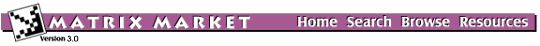# Browse the Harwell-Boeing Collection

Sets:
• ACOUST Dynamic analysis in structural engineering
• AIRTFC Air traffic control
• ASTROPH Astrophysics
• BCSPWR Power networks
• BCSSTRUC1 Dynamic analyses in structural engineering
• BCSSTRUC2 Static analyses in structural engineering
• BCSSTRUC3 Dynamic analyses in structural engineering
• BCSSTRUC4 Dynamic analyses in structural engineering.
• BCSSTRUC5 Structural engineering
• BCSSTRUC6 Structural engineering
• CANNES Finite-element structures problems in aircraft design.
• CEGB Finite-element calculations in structural engineering
• CHEMIMP Chemical engineering
• CHEMWEST Chemical engineering
• CIRPHYS Circuit physics
• COUNTERX Simple counter examples to Hellerman and Rarick algorithm
• DWT Structural engineering
• ECONAUS Economic modeling.
• ECONIEA Economic modeling.
• FACSIMILE Chemical kinetics
• GEMAT Power flow modeling
• GRENOBLE Simulation studies in computer systems.
• JAGMESH Finite-element model problem
• LANPRO Linear equations in structural engineering
• LAPLACE Partial differential equations
• LAPU Model finite-element calculations
• LNS Fluid flow modeling
• LOCKHEED Finite-element calculations in structural engineering
• LSHAPE Finite-element model problem
• LSQ Surveying
• MANTEUFFEL Finite-element calculations in structural engineering
• NNCENG Flow in networks
• NUCL Nuclear reactor modeling
• OILGEN Oil reservoir simulation
• PLATZ Oceanic modeling
• PORES Reservoir modeling